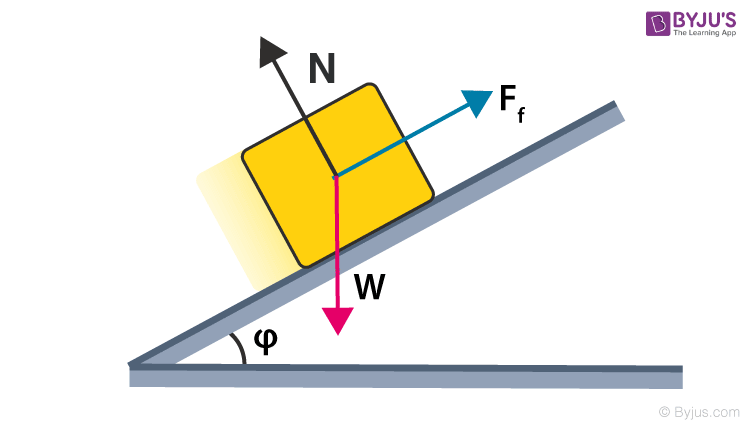Checkout JEE MAINS 2022 Question Paper Analysis : Checkout JEE MAINS 2022 Question Paper Analysis :

# Sliding Friction

## What is Sliding Friction?

We can define sliding friction as the resistance created by any two objects when sliding against each other. This friction is also known as kinetic friction and is defined as the force that is needed to keep a surface sliding along another surface. It depends on two variables- one is material and the other is the weight of the object. Any change in the surface area in contact does not change the sliding friction. In most of the materials, sliding friction is less than static friction. There are exceptions that include metals having static and sliding friction coefficients and are essentially the same with small surfaces where molecular attraction forces take over.### Sliding Friction Formula

The equation for sliding force includes the coefficient of sliding friction times the normal force.

FS = μSFn

Where,

FS =  force of sliding  friction

μS = Coefficient of sliding friction

Fn = normal force

### Factors affecting sliding friction

• The surface deformation of objects.
• The roughness or smoothness of the surface of the objects.
• The original speed of either object.
• The size of the object.
• Finally, the amount of pressure on either object.

### Examples of Sliding Friction

• Rubbing both the hands together to create heat.
• A child sliding down through a slide in a park.
• A coaster sliding against a table.
• A washing machine pushed along with the floor.
• The frame and the edge of the door sliding against one another.
• A block being slid across the floor.
• Two cards in a deck sliding against each other.

Sliding can occur between two objects of arbitrary shape whereas the rolling friction is the frictional force that is associated with the rotational movement. The rolling friction is usually less than the one associated with sliding kinetic friction. The values for the coefficient of rolling friction are quite less than that of sliding friction. It usually produces greater sound and thermal bi-products. For example- Movement of braking motor vehicle tires on a roadway.

## Frequently Asked Questions – FAQs

### What is sliding friction?

The force needed to keep a surface sliding along another surface is known as sliding friction.

### What are the two conditions on which sliding friction depends?

Sliding friction depends on the type material and weight of the object.

FS = μSFn

### Give two examples of sliding friction.

Examples of sliding friction are:
• A coaster sliding against a table.
• A block being slid across the floor.
• ### Does roughness or smoothness of the surface of the objects affect the sliding friction?

Yes sliding friction depends on the roughness or smoothness of the surface of the objects.
Test your Knowledge on Sliding Friction